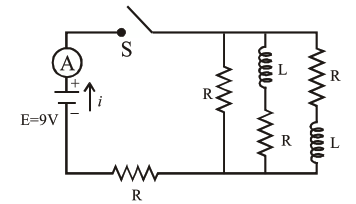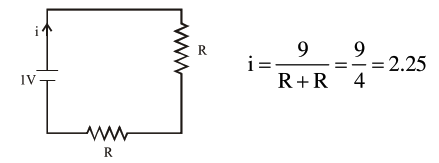# Figure shows a circuit thatQuestion:

Figure shows a circuit that contains four identical resistors with resistance $\mathrm{R}=2.0 \Omega$, two identical inductors with inductance $\mathrm{L}=2.0 \mathrm{mH}$ and an ideal battery with emf $\mathrm{E}=9 \mathrm{~V}$. The current ' $i$ ' just after the switch 'S' is closed will be :1. $2.25 \mathrm{~A}$

2. $3.0 \mathrm{~A}$

3. $3.37 \mathrm{~A}$

4. $9 \mathrm{~A}$

Correct Option: 1

Solution:

Just after the switch is closed, inductor will behave like infinite resistance (open circuit) so the circuit will look likeOption (1) is correct.